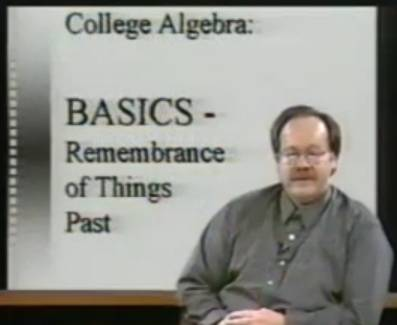# InfoCoBuild

## Math 110 - College AlgebraThis is a collection of video lectures for Math 110: College Algebra from UMKC (University of Missouri-Kansas city). Taught by Professor Richard Delaware, this course emphasizes the concepts of college algebra, covering a variety of topics on algebra: numbers, graphs, equations and inequalities, functions, polynomials, exponential and logarithmic functions, systems of linear equations, binomial theorem, and sequences.

More details of the videos are found:
Listing of the videos and their contents

### Unit 0 - Basics: Remembrance of Things Past

Lecture 01 - Numbers
Sets of Objects. Natural Numbers. Integers. Rational Numbers. Irrational Numbers. Real Numbers. The Real Line: From Numbers to Points. Real Numbers are Ordered. The Real Line: Distance Between Points. Real Numbers as Decimals

Lecture 02 - Language of Mathematics
Learning to Read Mathematics. Some Symbols of Algebra. Nouns, Pronouns, & The Main Verb of Algebra. Theorems, Corollaries, Lemmas, and All That

Lecture 03 - The Powers That be: Exponents
Integer Exponents. Operations with Integer Exponents. Square Roots: A Pair Of Equal Factors. Nth Roots & Rational Exponents. Operations with Rational Exponents

Lecture 4a - Polynomial Expressions
What is a Polynomial? Adding & Subtracting Polynomials. Multiplying Polynomials. A Common Error. Handy Polynomial Products. Un-Multiplying (Factoring) Polynomials. Completing A Perfect Square

Lecture 4b - Polynomial Expressions (cont.)
Dividing Polynomials: Rational Expressions. The Art of Simplification. Solving Some Polynomial & Rational Equations

Lecture 05 - More Numbers and a Little Geometry
Beyond Real Numbers: Complex Numbers. Some Area Formulas. The Pythagorean Theorem & A Visual Proof

### Unit 1 - Graphs

Lecture 06 - Graphs
Rectangular Coordinates: Geometry Meets Algebra. Distance Between Points. Midpoint of a Line Segment. Your Graphing Device

Lecture 07 - Graphs (cont.)
Graphs of Equations. Intercepts: Crossing the Axes. Symmetry of Graphs. Lines: Defining "Slope"

Lecture 08 - Graphs (cont.)
Lines & Their Equations. Parallel & Perpendicular Lines. Circles & Their Equations. Some Exercises Explained

### Unit 2 - Functions and Their Graphs

Lecture 09 - Functions and Their Graphs
Function: The Central Idea of Mathematics. The Language & Notation of Functions. More On Domains. Function Notation Practice

Lecture 10 - Functions and Their Graphs (cont.)
Visualizing Functions: Graphs of (x, f(x)) Pairs. Increasing & Decreasing Functions. Local Maximums & Local Minimums. Even & Odd Functions

Lecture 11 - Functions and Their Graphs (cont.)
A Library of Important Functions. Piecewise Defined Functions. Some Exercises Explained

Lecture 12 - Functions and Their Graphs (cont.)
Graphing Techniques: Vertical & Horizontal Shifts. Graphing Techniques: Compressions & Stretches. Graphing Techniques: Reflections Across the Axes. Putting It All Together: Moving The Graphs of Functions Around The Plane

Lecture 13 - Functions and Their Graphs (cont.)
The Algebra of Functions. A New Operation Unique To Functions: Composition

Lecture 14 - Functions and Their Graphs (cont.)
Mathematical Models of Real World Problems: Constructing Functions

### Unit 3 - Equations and Inequalities

Lecture 15 - Equations in One Variable
Solving Equations (Approximately) with a Graphing Device & The Intermediate Value Theorem. Solving Linear Equations: The "Linear Formula" or, Graphing. Solving Non-Linear Equations That Lead To Linear Equations

Lecture 16 - Equations in One Variable (cont.)
Solving Quadratic Equations: Factoring, or, Graphing. A Complex Reminder & The Principal Square Root of a Negative Number. Solving Quadratic Equations: The "Quadratic Formula" & The Discriminant or, Graphing

Lecture 17 - Equations in One Variable (cont.)
Some Linear & Quadratic Equation Exercises Explained

Lecture 18 - Equations in One Variable (cont.)
Setting Up Equations: More Mathematical Models

Lecture 19 - Equations in One Variable (cont.)

Lecture 20 - Inequalities in One Variable
Properties of Inequalities. Solving Inequalities In General. Solving Linear Inequalities

Lecture 21 - Inequalities in One Variable (cont.)
Solving Quadratic Inequalities. Solving Higher-Degree Polynomial Inequalities. Solving Rational Inequalities

Lecture 22 - Inequalities in One Variable (cont.)
When Absolute Value Appears: Equations. When Absolute Value Appears: Inequalities. More Exercises Explained

### Unit 4 - Polynomial and Rational Functions

Lecture 23 - Polynomial and Rational Functions

Lecture 24 - Polynomial and Rational Functions (cont.)
Degree "n": General Polynomial Functions. Special Case: Power Functions & Their Graphs. Graphing General Polynomial Functions: Zeros, Multiplicity, Turning Points & End Behavior

Lecture 25 - Locating the Zeros of a Polynomial Function
How Many Zeros Are There? How Many Zeros Are Real? How Many Real Zeros Are Positive? Negative? Where (On What Interval) Are All Real Zeros? How Can You Guess The Location of Real Zeros? How Can You Reduce The Number of Real Zeros?

Lecture 26 - Locating the Zeros of a Polynomial Function (cont.)
Strategy & Tools: A Practical Checklist. Some Polynomial Exercises Explained

Lecture 27 - Rational Functions
General Rational Functions. What Is An Asymptote? Finding Asymptotes of Rational Functions. Graphing Rational Functions

### Unit 5 - Exponential and Logarithmic Functions

Lecture 28 - Exponential Functions
One-To-One Functions. Exponential Functions & Their Graphs. The Natural Exponential Function

Lecture 29 - Logarithmic Functions
Inverse Functions. Logarithmic Functions & Their Graphs. The Natural Logarithmic Function

Lecture 30 - Logarithmic Functions (cont.)
Properties of Logarithms. All Logarithms are Natural (or Common)! Solving Logarithmic Equations. Logarithmic Models: Sound (Loudness) & Fury (Earthquakes)

Lecture 31 - Exponential Functions (cont.)
Solving Exponential Equations. Exponential Models: Compounded Interest, and Growth & Decay

### Unit 6 - Systems of Equations

Lecture 32 - Systems of Linear Equations
Systems of Linear Equations in General. Solving A System of 2 (or 3) Linear Equations in 2 (or 3) Variables: Substitution. Solving A System of 2 (or 3) Linear Equations in 2 (or 3) Variables: Elimination

Lecture 33 - Systems of Linear Equations (cont.)
Some Exercises Explained. An Application: Writing Proper Rational Functions As Sums of Simpler Proper Rational Functions (Partial Fractions)

Lecture 34 - Systems of Non-Linear Equations
Solving (Mostly Graphically) a System of 2 Non-Linear Equations in 2 Variables

### Unit 7 - Some Discrete Topics

Lecture 35 - Sequences
Infinite Sequences: Functions With Domain N. The Factorial Symbol: !. Adding The First n Terms of a Sequence: nth Partial Sums & Summation Notation

Lecture 36 - Sequences (cont.)